# waynehu

## Professor, Department of Astronomy and Astrophysics University of Chicago

Group Contact CV SnapShots

## Bandpower Estimation

Figure 5 indicates that the next step in the compression process is extracting bandpowers from the map. What is a bandpower and how can it be extracted from the map? To answer these questions, we must construct a new likelihood function, one in which the estimated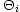are the data. No theory predicts an individual, but all predict the distribution from which the individual temperatures are drawn. For example, if the theory predicts Gaussian fluctuations, thenis distributed as a Gaussian with mean zero and covariance equal to the sum of the noise covariance matrixand the covariance due to the finite sample of the cosmic signal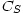. Inverting Equation (1) and using Equation (2) for the ensemble average leads to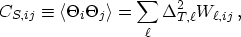(30)

where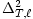depends on the theoretical parameters through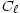(see Equation (3)). Here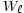, the window function, is proportional to the Legendre polynomial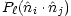and a beam and pixel smearing factor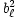. For example, a Gaussian beam of width, dictates that the observed map is actually a smoothed picture of true signal, insensitive to structure on scales smaller than. If the pixel scale is much smaller than the beam scale,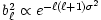. Techniques for handling asymmetric beams have also recently been developed [Wu et al, 2001,Wandelt & Gorski, 2001,Souradeep & Ratra, 2001]. Using bandpowers corresponds to assuming thatis constant over a finite range, or band, of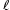, equal to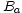for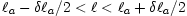. Plate 1 gives a sense of the width and number of bands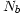probed by existing experiments.

For Gaussian theories, then, the likelihood function is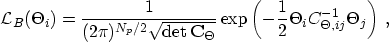(31)

where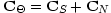and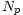is the number of pixels in the map. As before,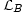is Gaussian in the anisotropies, but in this caseare not the parameters to be determined; the theoretical parameters are the, upon which the covariance matrix depends. Therefore, the likelihood function is not Gaussian in the parameters, and there is no simple, analytic way to find the point in parameter space (which is multi-dimensional depending on the number of bands being fit) at whichis a maximum. An alternative is to evaluatenumerically at many points in a grid in parameter space. The maximum of theon this grid then determines the best fit values of the parameters. Confidence levels on say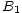can be determined by finding the region within which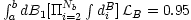, say, for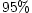limits.

This possibility is no longer viable due to the sheer volume of data. Consider the Boomerang experiment with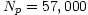. A single evaluation ofinvolves computation of the inverse and determinant of the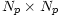matrix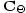, both of which scale as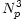. While this single evaluation might be possible with a powerful computer, a single evaluation does not suffice. The parameter space consists of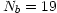bandpowers equally spaced from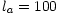up to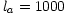. A blindly placed grid on this space would require at least ten evaluations in each dimension, so the time required to adequately evaluate the bandpowers would scale as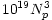. No computer can do this. The situation is rapidly getting worse (better) since Planck will have of order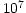pixels and be sensitive to of order a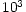bands.

It is clear that a smart'' sampling of the likelihood in parameter space is necessary. The numerical problem, searching for the local maximum of a function, is well-posed, and a number of search algorithms might be used.tends to be sufficiently structureless that these techniques suffice. [Bond et al, 1998] proposed the Newton-Raphson method which has become widely used. One expands the derivative of the log of the likelihood function - which vanishes at the true maximum of- around a trial point in parameter space,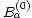. Keeping terms second order in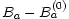leads to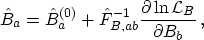(32)

where the curvature matrixis the second derivative of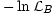with respect toand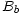. Note the subtle distinction between the curvature matrix and the Fisher matrix in Equation (29),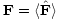. In general, the curvature matrix depends on the data, on the. In practice, though, analysts typically use the inverse of the Fisher matrix in Equation (32). In that case, the estimator becomes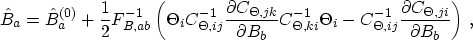(33)

quadratic in the data. The Fisher matrix is equal to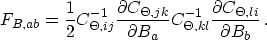(34)

In the spirit of the Newton-Raphson method, Equation (33) is used iteratively but often converges after just a handful of iterations. The usual approximation is then to take the covariance between the bands as the inverse of the Fisher matrix evaluated at the convergent point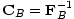. Indeed, [Tegmark, 1997b] derived the identical estimator by considering all unbiased quadratic estimators, and identifying this one as the one with the smallest variance.

Although the estimator in Equation (33) represents a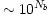improvement over brute force coverage of the parameter space - converging in just several iterations - it still requires operations which scale as. One means of speeding up the calculations is to transform the data from the pixel basis to the so-called signal-to-noise basis, based on an initial guess as to the signal, and throwing out those modes which have low signal-to-noise [Bond, 1995,Bunn & Sugiyama, 1995]. The drawback is that this procedure still requires at least oneoperation and potentially many as the guess at the signal improves by iteration. Methods to truly avoid this prohibitivescaling [Oh et al, 1999,Wandelt & Hansen, 2001] have been devised for experiments with particular scan strategies, but the general problem remains open. A potentially promising approach involves extracting the real space correlation functions as an intermediate step between the map and the bandpowers [Szapudi et al, 2001]. Another involves consistently analyzing coarsely pixelized maps with finely pixelized sub-maps [Dore et al, 2001].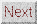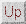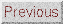Next: Cosmological Parameter Estimation Up: DATA ANALYSIS Previous: Mapmaking
Wayne Hu 2001-10-15